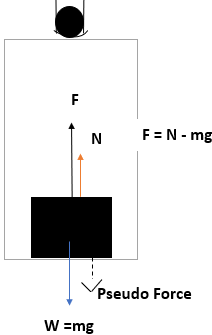# If a block is kept in an accelerating elevator, then how does the pseudo force generated act, and...

## Question:

If a block is kept in an accelerating elevator, then how does the pseudo force generated act, and on which body does it act?

## Concept of Pseudo Force:

The frame of reference is the imaginary system confining the observable like the initial and final velocity of the body, time, etc. The frame of reference can inertial and non-inertial frames of reference.

• The pseudo can be related to the action of inertia.
• Whenever the frame of reference is subjected to the acceleration then the body tends to conserve its state of rest/motion.
• This opposing effect by the body in a frame of reference generates the pseudo force.
• For the case of a block lying on the floor of a lift will have weight force acting on it due to gravity.
• As a result of weight force, the normal force will act in the upward direction.
• The system of frame of reference in the aforesaid condition is inertial frame of reference (Newtonian mechanics is applicable).
• Now for the case when the lift accelerates upwards then the resultant force is in the upward direction.
• The inertia will tend the body to remain at rest (initially) and they tend to keep the body at uniform velocity every time when the lift speed is increasing.
• Therefore, a continuous pseudo force would be acting on the block in addition to its weight force (in a downward direction).
• Hence, the block would appear heavier when the elevator is accelerating upwards.
• The case reverses when the elevator is accelerating downwards and the block would appear lighter.

The diagram is shown below,